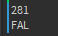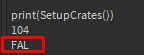# Randomizer always returning same object/name

Hello, so I made a post a while ago, about how to make well, randomizer, the funny part, is that, it’s always returning “FAL” for some reason, even when sum is more than 10, which is pretty weird, any idea of how to fix?
OUTPUT:``````local CrateItems = {
{Name="FAL", RandomNumber=10, Type="Primary"},
{Name="AK74M", RandomNumber=30, Type="Primary"},
{Name="MP9", RandomNumber=70, Type="Primary"},
{Name="Desert Eagle", RandomNumber=50, Type="Secondary"},
{Name="Micro UZI", RandomNumber=70, Type="Secondary"},
{Name="USP", RandomNumber=100, Type="Secondary"},
{Name="Nothing!", RandomNumber=100, Type="NIL"},
}

local function DevolveSuma()
local sum = 0
for i,v in ipairs(CrateItems) do
if v then
sum = sum + v.RandomNumber
end
end
return sum
end

local function SetupCrates()
local RandomNumber = math.random(DevolveSuma())
for i,v in ipairs(CrateItems) do
if v.RandomNumber <= RandomNumber then
print(RandomNumber)
return v.Name
end
end
end

print(SetupCrates())
``````
1 Like

`DevolveSuma` is returning 430, so `RandomNumber` is from 1 to 430. Then in the loop, the FAL is the first index in `CrateItems` and gets checked first against Random number. Because RandomNumber is most likely >= 10, the loop usually returns after the FAL.

2 Likes

What do you mean?
Because if loop is >= 10 and I’m checking if fal RandomNumber is <= TheRandomNumber
It shouldn’t be printing fal because RandomNumber is >= of fal? What can I do to fix it

FAL.RandomNumber is 10. RandomNumber is between 1 and 430. Therefore, it is likely true that FAL.RandomNumber <= RandomNumber.

So then can I fix it, and how is printing “FAL” if the RandomNumber is <= the fal random number? Lol.
How can I fix itYes, because randomness are generated by a seed. These seed will always stay the same, so you want to change it everytime. To fix this, you usually would set the random seed to something that changes every time like os.time.

What do you mean?
I set randomseed to tick() and still doesn’t works.

``````local function SetupCrates()
math.randomseed(tick())
local RandomNumber = math.random(DevolveSuma())
for i,v in ipairs(CrateItems) do
if v.RandomNumber <= RandomNumber then
print(RandomNumber)
return v.Name
else
RandomNumber = RandomNumber - v.RandomNumber
end
end
end

``````

But how it’s printing fal if the RandomNumber is more than v.RandomNumber

The conditional here is the issue, as @AstroCode pointed out.

You have to change the conditional so it checks the chosen number is in the right range, and for that you also need another variable to track the previous numbers. Here’s that solution:

``````local function SetupCrates()
local RandomNumber = math.random(DevolveSuma())
local soFar = 0
for i,v in ipairs(CrateItems) do
if RandomNumber > soFar and RandomNumber <= soFar + v.RandomNumber then --Is above the minimum and below or equal to the maximum
return v.Name
end
soFar = soFar + v.RandomNumber
end
end
``````

If you have any questions about how this works, feel free to ask them.

1 Like